### Home > MC2 > Chapter Ch7 > Lesson 7.1.5 > Problem7-52

7-52.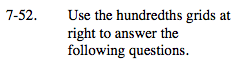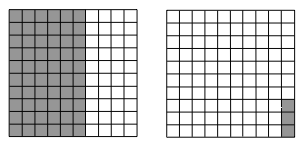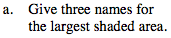Find the number of shaded squares by finding the area represented or by counting each square. If this number is out of 100 total squares, how can you represent this value as a fraction, decimal, or percentage?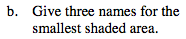See part (a).

$0.03 = 3\%= \frac{3}{100}$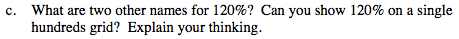How can 120% be represented as a fraction or decimal value?

If there are only 100 squares on a single grid, can you represent 120 squares on this grid?